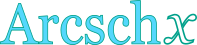## Inverse hyperbolic cosecant calculator

This tool evaluates the inverse hyperbolic cosecant of a number: arcsch(x). Enter the argument x below.

 x = Result: arcsch(x) =## Definitions

### General

The inverse hyperbolic cosecant function, in modern notation written as arcsch(x) or arccsch(x) or csch-1x, gives the value t (hyperbolic angle), so that:

The inverse hyperbolic cosecant function accepts as arguments all real numbers except zero. Since the hyperbolic cosecant is defined through the natural exponential function , its inverse can be defined through the natural logarithm function, using the following formula, for real x, with x≠1:

### Properties

The derivative of the inverse hyperbolic cosecant function is:

The integral of the inverse hyperbolic cosecant function is given by: